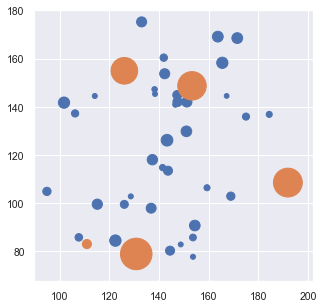# Notebook 5.4 - Capacitated Facility Network Location Problem¶

In :
import numpy as np
import matplotlib
import matplotlib.pyplot as plt
import seaborn as sns; sns.set()
from sklearn.datasets import make_blobs

%matplotlib inline

plot_size   = 14
plot_width  = 5
plot_height = 5

params = {'legend.fontsize': 'large',
'figure.figsize': (plot_width,plot_height),
'axes.labelsize': plot_size,
'axes.titlesize': plot_size,
'xtick.labelsize': plot_size*0.75,
'ytick.labelsize': plot_size*0.75,
plt.rcParams.update(params)

In :
import os

os.system('jupyter nbconvert --to html yourNotebook.ipynb')

Out:
-1
In :
center_box = (100, 200)

n_customer = 40
n_facility = 5

facil_cap_low  = 400
facil_cap_high = 1200

custm_dem_low  = 30
custm_dem_high = 80

transp_unit_cost = 2
capacity_unit_cost = 100


For this problem we are going to be randomly generating quite a few values to complement our dataset of customers and facilities. These are defined in the cell above.

In :
np.random.seed(2)


We are using a random number generator to populate the values for facility capacities and customer demands. If we want to ensure that we always get the same instance if we rerun the notebook, we can set a random seed (here: 2) using the command above.

Different seed values will result in differnt datasets.

In :
facil_capacities = np.random.randint(low=facil_cap_low,
high=facil_cap_high,
size=n_facility)

facil_capacities

Out:
array([568, 927, 893, 984, 934])

The np.random.randint will create an array of random integers (here, of size n_facility), between the values defined by the low and high parameters.

In :
custm_demands = np.random.randint(low=custm_dem_low,
high=custm_dem_high,
size=n_customer)

In :
print(f'sum_facil ({sum(facil_capacities)}) > sum_cust ({sum(custm_demands)}) = {sum(facil_capacities) > sum(custm_demands)})')

sum_facil (4306) > sum_cust (2222) = True)


As a sanity check, we are testing whether we have enough facility capacity to serve our customers.

In :
cust_coord , _ = make_blobs(n_samples=n_customer,
centers=2,
cluster_std=20,
center_box=center_box,
random_state =  2)

facil_coord, _ = make_blobs(n_samples=n_facility,
centers=1,
cluster_std=30,
center_box=center_box,
random_state = 50)

In :
custm_node_size = plot_size*10*((custm_demands/custm_dem_high)**2)
facil_node_size = plot_size*300*(((facil_capacities-facil_cap_low)/facil_cap_high)**2)

plt.scatter(cust_coord[:, 0],
cust_coord[:, 1],
s=custm_node_size,
cmap='viridis',
zorder=1500);

plt.scatter(facil_coord[:, 0],
facil_coord[:, 1],
s=facil_node_size,
cmap='viridis',
zorder=2000);We are using nodes of varying sizes to illustrate the differences in capacities and demands. Adjust the parameters as necessary.

In :
from scipy.spatial import distance

dist_matrix = distance.cdist(cust_coord, facil_coord, 'euclidean')